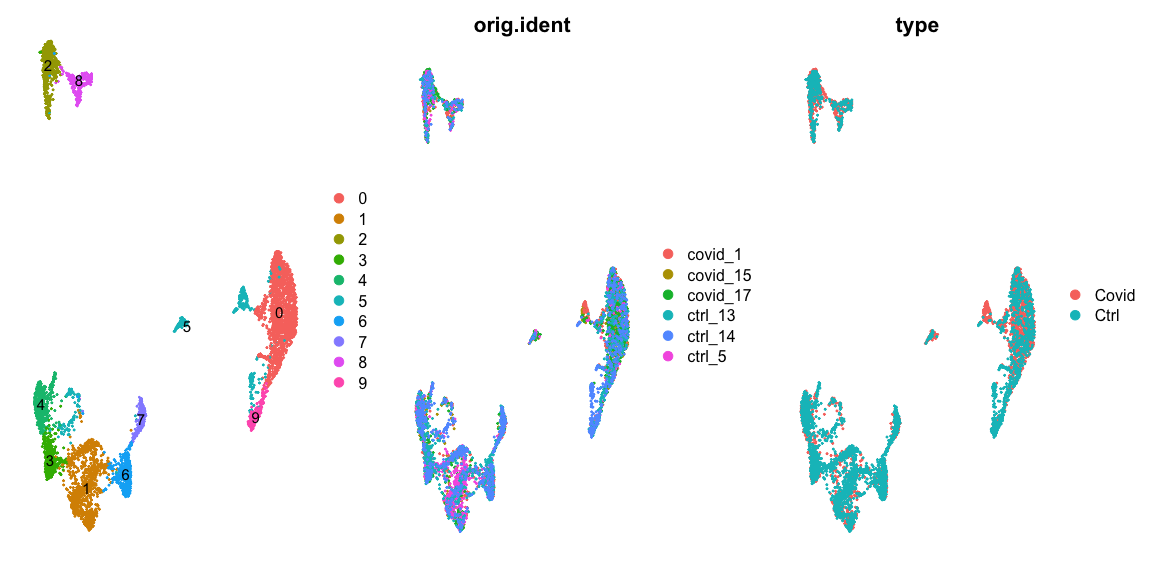# Differential gene expression

In this tutorial we will cover about Differetial gene expression, which comprises an extensive range of topics and methods. In single cell, differential expresison can have multiple functionalities such as of identifying marker genes for cell populations, as well as differentially regulated genes across conditions (healthy vs control). We will also exercise on how to account the batch information in your test.

We can first load the data from the clustering session. Moreover, we can already decide which clustering resolution to use. First let’s define using the `louvain` clustering to identifying differentially expressed genes.

``````suppressPackageStartupMessages({
library(Seurat)
library(dplyr)
library(cowplot)
library(ggplot2)
library(pheatmap)
library(enrichR)
library(rafalib)
library(Matrix)
library(edgeR)
library(MAST)
})

``````# Set the identity as louvain with resolution 0.5
sel.clust = "CCA_snn_res.0.5"

alldata <- SetIdent(alldata, value = sel.clust)
table(alldata@active.ident)``````
``````##
##    0    1    2    3    4    5    6    7    8    9
## 1536 1014  568  442  427  418  407  268  242  210``````
``````# plot this clustering
plot_grid(ncol = 3, DimPlot(alldata, label = T) + NoAxes(), DimPlot(alldata, group.by = "orig.ident") +
NoAxes(), DimPlot(alldata, group.by = "type") + NoAxes())``````## Cell marker genes

Let us first compute a ranking for the highly differential genes in each cluster. There are many different tests and parameters to be chosen that can be used to refine your results. When looking for marker genes, we want genes that are positivelly expressed in a cell type and possibly not expressed in the others.

``````# Compute differentiall expression
markers_genes <- FindAllMarkers(alldata, log2FC.threshold = 0.2, test.use = "wilcox",
min.pct = 0.1, min.diff.pct = 0.2, only.pos = TRUE, max.cells.per.ident = 50,
assay = "RNA")``````

We can now select the top 25 up regulated genes for plotting.

``````markers_genes %>%
group_by(cluster) %>%
``````mypar(2, 5, mar = c(4, 6, 3, 1))